Mobile QR CodeTFET, vertical structure TFET, AC switching characteristics of TFET

## I. INTRODUCTION

For the low power consumption of metal-oxide-semiconductor field-effect transistors (MOSFETs), supply voltage (V$_{\mathrm{DD}}$) needs to be scaled down. Furthermore, for maintaining high on-current (I$_{\mathrm{ON}}$) despite of the V$_{\mathrm{DD}}$ reduction, extremely low subthreshold swing (SS) is necessary. However, it is well-known that the SS of a MOSFET cannot be lowered below 60 mV/dec at room temperature because its operation is based on thermionic carrier injection (1). A tunneling field-effect transistor (TFET) has been regarded as one of the most promising candidates for a next-generation low-power device due to its low SS (sub-kT/q SS at room temperature) and excellent complementary MOS (CMOS) process compatibility (2-4). However, there are still a lot of challenges in the way to commercialization: particularly, ambipolar current by undesirable drain-side tunneling and low I$_{\mathrm{ON}}$.

In order to overcome these disadvantages, TFETs with elevated drain (TFET$_{\mathrm{ED}}$) have been proposed since TFET$_{\mathrm{ED}}$ not only reduces the tunneling resistance by using smaller band-gap materials such as SiGe or Ge as a channel, but also suppresses the ambipolar current by forming the Si elevated drain on the channel (5) (TFET$_{\mathrm{ED}}$ is one of the TFETs with vertical structures. In this study, TFET$_{\mathrm{ED}}$ is used to represent the TFETs with various vertical structures). Though TFETs with vertical structures (TFET$_{\mathrm{VS}}$) have been reported to suppress the ambipolar current and to enhance the I$_{\mathrm{ON}}$, the previous works on the TFET$_{\mathrm{VS}}$s have mainly focused on tunneling dynamics between source and channel. In this study, the effects of various pillar conditions such as pillar thickness (T$_{\mathrm{pillar}}$), pillar height (T$_{\mathrm{Si}}$), the ratio of channel length and pillar height, and the doping concentration of pillar on DC/AC characteristics of TFET$_{\mathrm{VS}}$s are rigorously investigated through mixed-mode device and circuit TCAD simulations.

Fig. 1. Device structure and physical parameters of TFETs used in the simulations.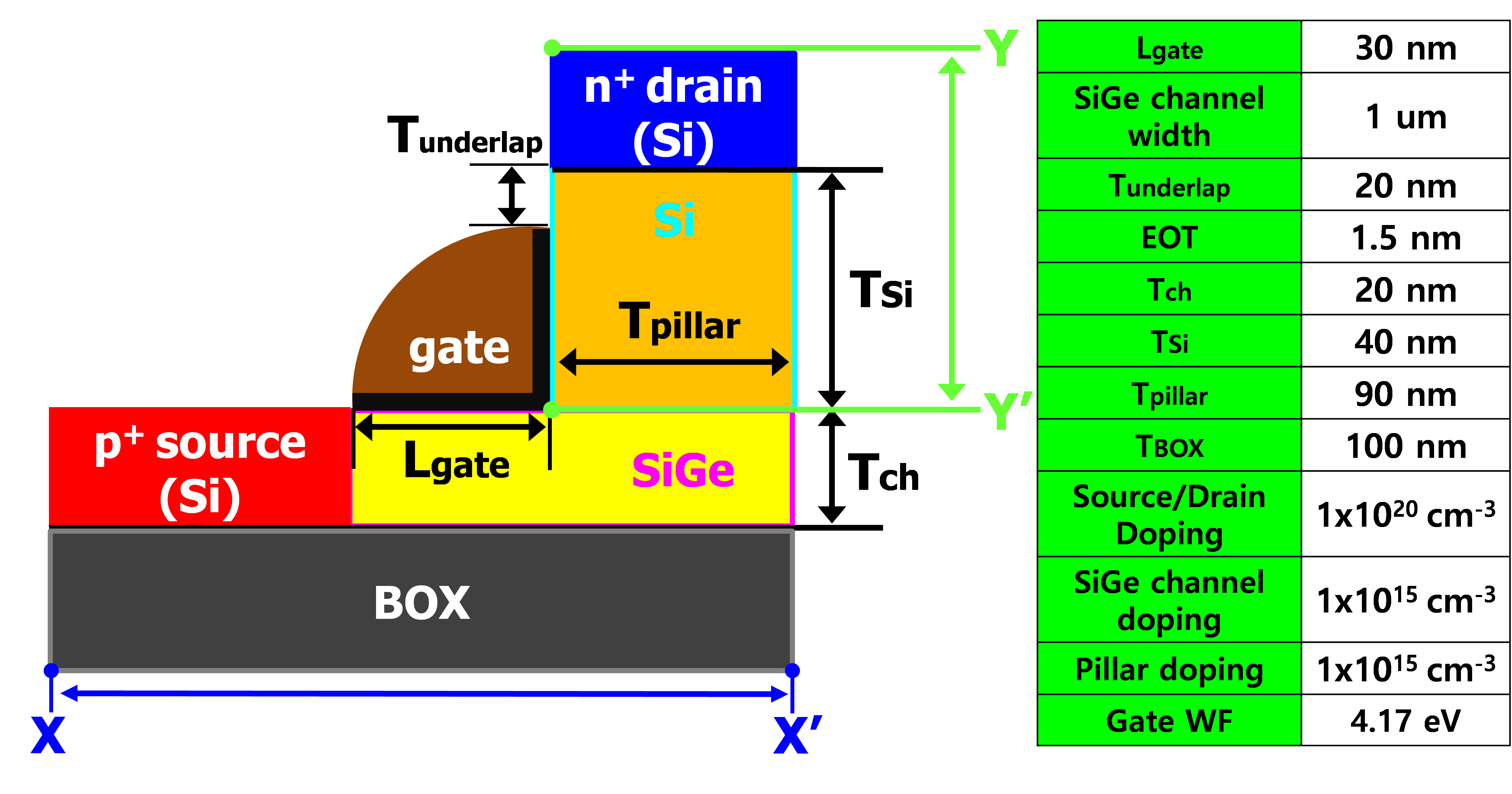## II. DEVICE STRUCTURE AND SIMULATION CONDITIONS

The cross-sectional structure of TFET$_{\mathrm{VS}}$s used in this simulation study is shown in Fig. 1. In order to analyze the characteristics of TFET$_{\mathrm{VS}}$s in an inverter configuration, mixed-mode device and circuit simulations are conducted by using Synopsys Sentaurus$^{\mathrm{TM}}$ which is especially suitable for accurate tunneling current calculation because it automatically defines tunneling path and mesh on the basis of the valence-band gradient (6-8). The table of Fig. 1 shows the device parameters used in the simulations where a uniform doping profile is used for all the regions (namely, the source, the channel, and the drain regions) with an abrupt profile at the interfaces as applicable between them. Although the artificial doping profiles may result in the some disagreement in the current levels, this does not have much impact on our findings as the focus of this study is not on the exact values of currents but more on the changes of the device characteristics and the inverter switching characteristics by various pillar conditions. N-type TFET (nTFET) consists of p$^{+}$ source, p$^{- }$intrinsic channel, and n$^{+}$ drain, while the p$^{+}$ source/p$^{- }$channel/n$^{+}$ drain are replaced by n$^{+}$ source/n$^{- }$channel/p$^{+}$ drain in p-type TFET (pTFET). Gate leakage current is ignored, Fermi statistics is assumed, and nonlocal band-to-band tunneling (BTBT), drift-diffusion carrier transport, band-gap narrowing and Shockley-Read-Hall (SRH) recombination models are used.

To reflect accurate tunneling currents, tunneling model is first calibrated to experimental data for fabricated Si and Si$_{\mathrm{0.7}}$Ge$_{\mathrm{0.3}}$ TFETs. The Si TFET is fabricated on a (100) p-type silicon-on-insulator (SOI) wafer with 100 nm Si thickness on top of 375 nm buried oxide. The gate stack consists of 200 nm poly-Si layer and 3 nm SiO$_{2}$. After gate patterning, source and drain regions are defined through photolithography and implantation processes. BF$_{2}$ with dose of 8${\times}$10$^{14}$ cm$^{-2}$, 7˚ tilt, and energy of 10 keV is used for source implantation. Drain implantation is also performed by using Arsenic (As) with the same condition. Dopant activation is implemented by rapid thermal process (RTP) at 900 $^{\circ}$C during 5 sec. Also, the SiGe TFET is fabricated on a (100) p-type SOI wafer with 60 nm Si thickness. Epitaxial growing of the SiGe with Ge content of 30 percent and thickness of 40 nm is performed on the SOI wafer. Then, Si capping layer is grown on the SiGe channel to reduce defects at the interface between gate stack and SiGe channel (The capping layer is completely consumed to SiO$_{2}$ after gate oxidation). The following fabrication processes are the same as those of the Si TFET.

Fig. 2. Calibration results of simulated trasfer characteristics at drain bias (V$_{\mathrm{D}}$) of 0.8 V. Tunneling model is calibrated to experimental data for the fabricated planar Si and SiGe TFETs.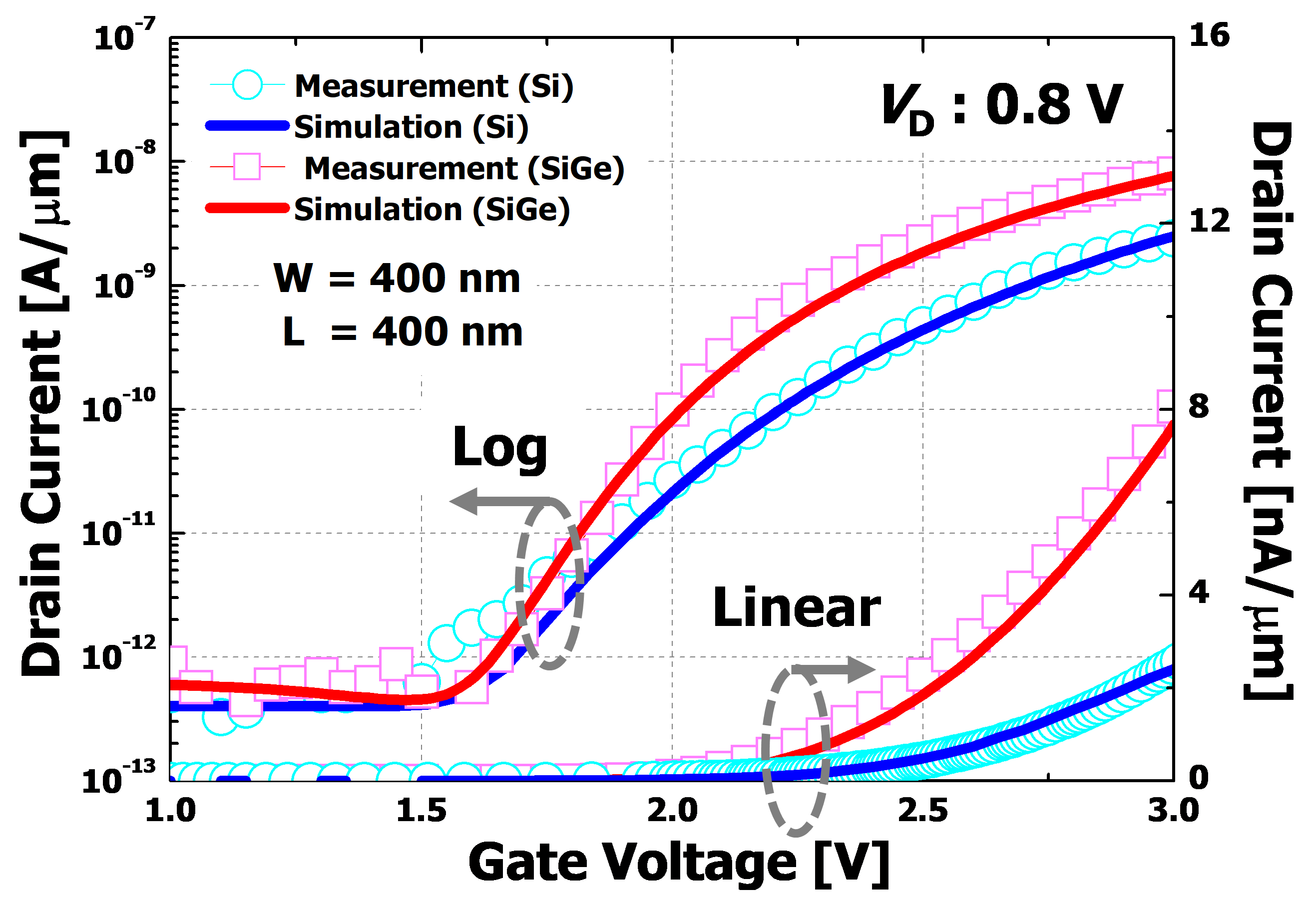To calculate BTBT generation rate (G) per unit volume in the uniform electric field, Kane’s model is used [Eq. (1)] (9) and fitted parameters are as follows.

##### (1)
\begin{equation} G=A\left(\frac{F}{F_{0}}\right)^{P}\exp \left(- \frac{B}{F}\right) \end{equation}

where F$_{0}$ = 1 V/m, P = 2.5 for indirect BTBT, A$_{\mathrm{Si}}$ = 4.0 ${\times}$ 10$^{14 }$/ A$_{\mathrm{SiGe}}$ = 3.1 ${\times}$ 10$^{16}$ cm$^{-1}$·s$^{-1}$ and B$_{\mathrm{Si}}$ = 9.9 ${\times}$ 10$^{6 }$/ B$_{\mathrm{SiGe}}$ = 7.1 ${\times}$ 10$^{5}$ are Kane parameters of experimentally calibrated Si and SiGe materials respectively, and F is the electric field. Fig. 2 shows that the calibration results of the simulated transfer curves are well fitted to the measured data of the fabricated TFETs. Then, transient responses of TFET$_{\mathrm{VS}}$ inverters with various pillar conditions are simulated for an input step voltage (V$_{\mathrm{in}}$) with a peak-to-peak voltage of 0.8 V and a rise/fall time of 1 ns as can be seen in Fig. 6(c). Here, V$_{\mathrm{DD}}$ = 0.8 V and load capacitance (C$_{\mathrm{L}}$) = 3 ${\times}$ 10$^{-15}$ F are used.

## III. RESULTS AND DISCUSSION

TCAD simulations are performed to investigate the effects of various pillar conditions on DC characteristics of TFET$_{\mathrm{VS}}$ devices and AC switching characteristics of TFET$_{\mathrm{VS}}$ inverters$_{\mathrm{.}}$ The device parameters of Fig. 1 are used in all the simulations except for changed conditions. Fig. 3(a) and (b) show that the drain current (I$_{\mathrm{D}}$) of TFET$_{\mathrm{VS}}$ becomes increased in both the transfer and output characteristics as the T$_{\mathrm{pillar}}$ gets thicker. This can be explained by the energy band diagrams of Fig. 4(a) and (b). The energy band diagrams show that gate voltage (V$_{\mathrm{G}}$) controllability on the drain-side channel becomes weaker and drain voltage (V$_{\mathrm{D}}$) has the stronger influence on the tunneling barrier between source and channel as the T$_{\mathrm{pillar}}$ gets thicker, similarly to drain-induced barrier lowering (DIBL) of MOSFETs. Thus, the tunneling current between source and channel gets increased due to the V$_{\mathrm{D}}$-induced shorter tunneling length with the thicker T$_{\mathrm{pillar}}$. To clarify the effects of the V$_{\mathrm{D}}$ on the source-side tunneling barrier and to optimize the pillar conditions, the pillar height (T$_{\mathrm{Si}}$) and the doping concentration of the Si pillar are changed respectively. Fig. 5(a) indicates that the I$_{\mathrm{D}}$ gets decreased as the Si pillar has the higher doping concentration (p-type dopants are applied) because the depletion region formed at the interface between the drain and the Si pillar is reduced and thus the influence of the V$_{\mathrm{D}}$ on the source-side tunneling barrier becomes weaker with the higher doping concentration. Also, Fig. 5(b) shows that the I$_{\mathrm{D}}$ gets decreased with the higher pillar since the V$_{\mathrm{D}}$ has the weaker influence on the source-side tunneling barrier by the increased distance between the source and the drain. Additionally, the length of the SiGe channel (L$_{\mathrm{gate}}$) is increased with the fixed pillar conditions of Fig. 1. Fig. 5(c) demonstrates that the I$_{\mathrm{D}}$ gets reduced as the L$_{\mathrm{gate}}$ becomes longer since the source is located further from the drain with the longer L$_{\mathrm{gate}}$ consistently with the results of the T$_{\mathrm{Si}}$ variation.

Fig. 3. (a) Transfer characteristics of n-type TFETs (nTFET) with various T$_{\mathrm{pillar}}$s. The inset shows the transfer characteristics of linear scale, (b) Output characteristics of nTFETs with various T$_{\mathrm{pillar}}$s.}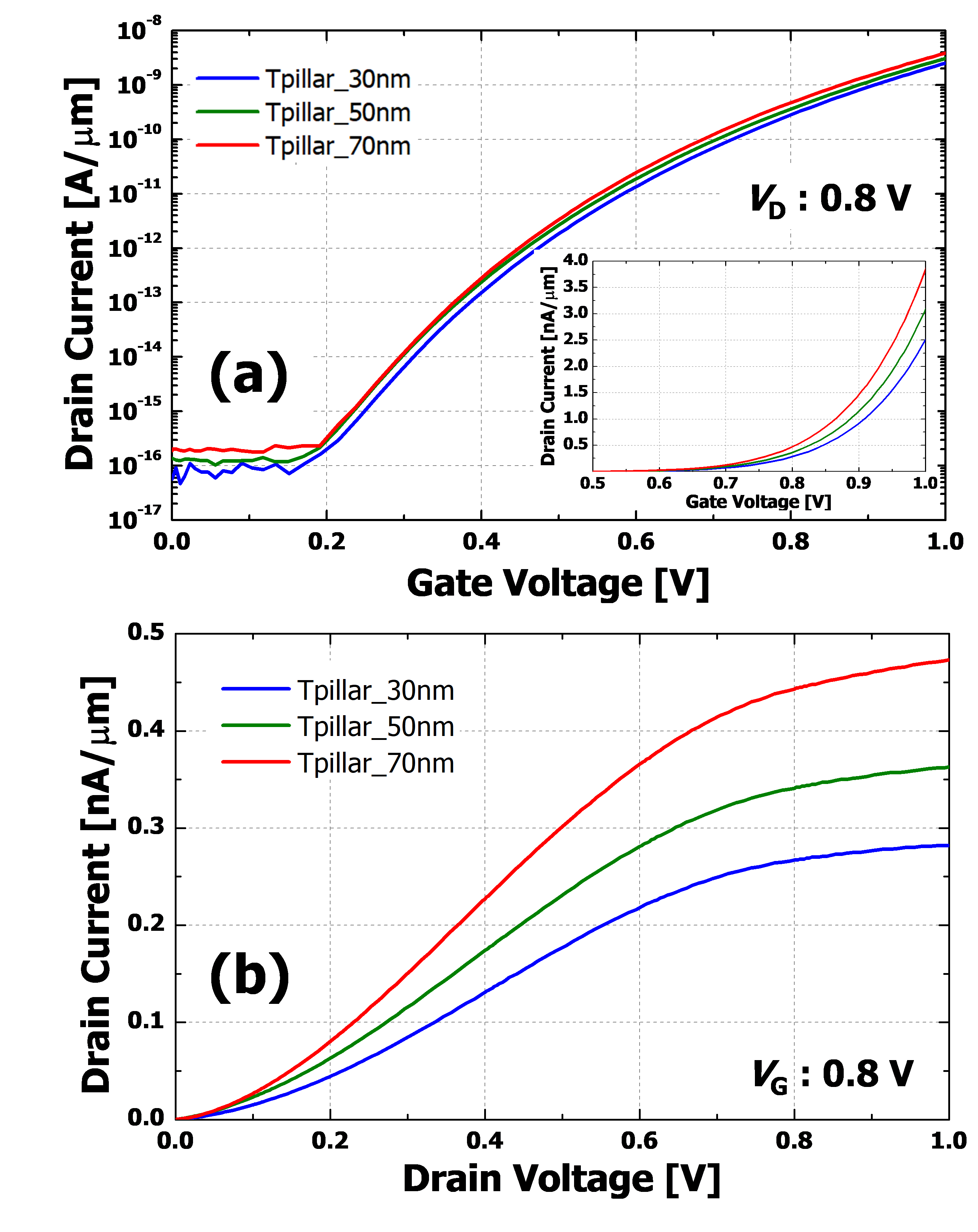Fig. 4. (a) Energy band diagrams between source and channel in nTFETs with various T$_{\mathrm{pillar}}$s, (b) Energy band diagrams between drain and channel in nTFETs with various T$_{\mathrm{pillar}}$s.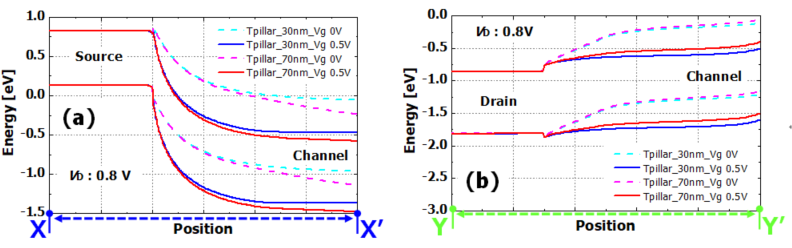Fig. 5. The changes of transfer characteristics as a function of (a) doping concentration of pillar, (b) pillar height (T$_{\mathrm{Si}}$), (c) length of SiGe channel (L$_{\mathrm{gate}}$).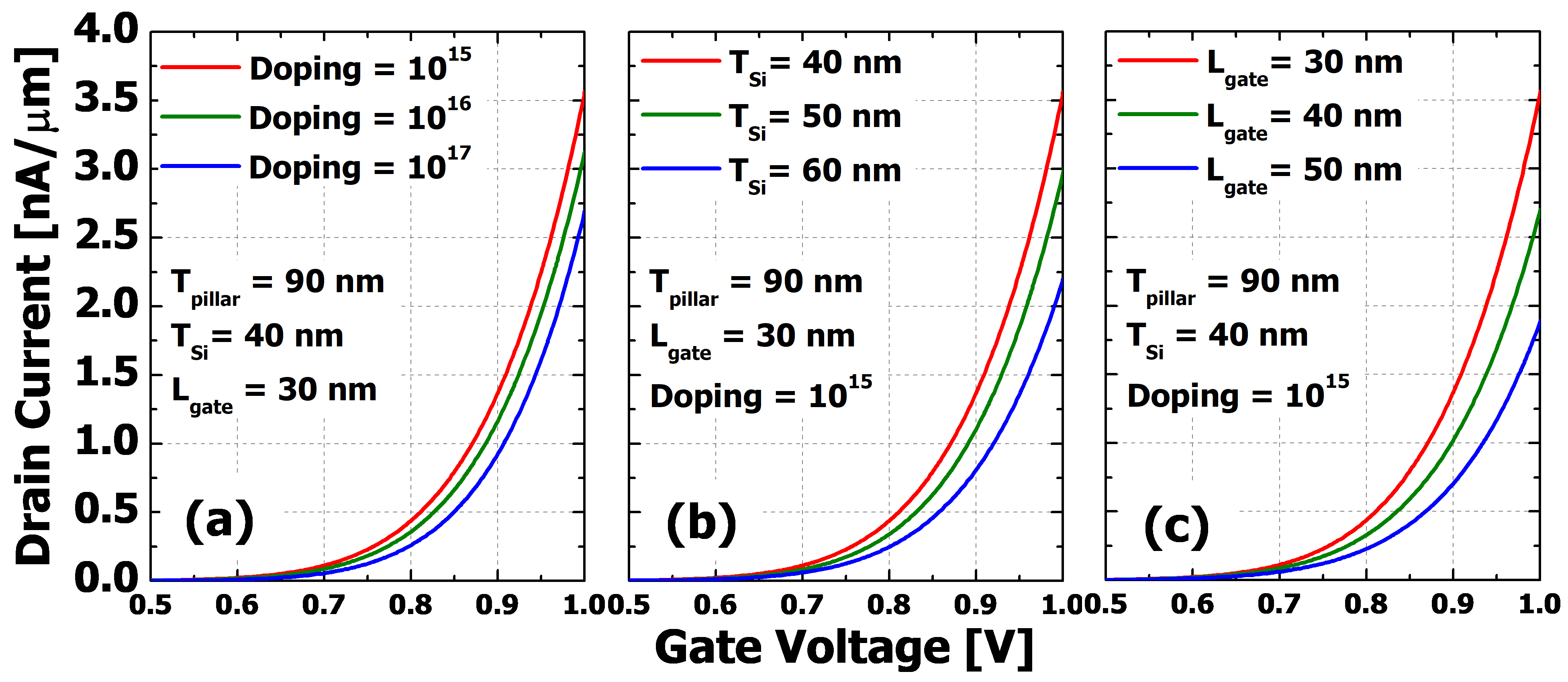Furthermore, the V$_{\mathrm{G}}$ controllability on the drain-side channel can be verified by gate-to-drain capacitance (C$_{\mathrm{GD}}$)-V$_{\mathrm{G}}$ characteristics of TFET$_{\mathrm{VS}}$. Fig. 6(a) shows that the C$_{\mathrm{GD}}$-V$_{\mathrm{G}}$ curve gets positive-shifted as the T$_{\mathrm{pillar}}$ becomes thicker (As an example of the V$_{\mathrm{G}}$ controllability, the effects of the Tpillar on the C$_{\mathrm{GD}}$-V$_{\mathrm{G}}$ curve are simulated.) This phenomenon can be explained as follows: For TFETs, the C$_{\mathrm{GD}}$ reflects the entire gate capacitance (C$_{\mathrm{GG}}$) when the drain quasi-Fermi energy is well above the conduction band edge of the channel (namely, the occurrence of the drain-side channel inversion) (10). As can be seen in the energy band diagrams of Fig. 4(b), the drain-side channel inversion occurs at larger V$_{\mathrm{G}}$ with the thicker T$_{\mathrm{pillar}}$ because the V$_{\mathrm{G}}$ has the weaker controllability on the drain-side channel by the thicker T$_{\mathrm{pillar}}$, which leads to the positive-shifted C$_{\mathrm{GD}}$-V$_{\mathrm{G}}$ curve. (Although both n-type and p-type TFETs are used for all the simulations, only the n-type branch is shown in the characteristics for simplicity.)

To investigate the effects of the increased I$_{\mathrm{D}}$ and the positive-shifted C$_{\mathrm{GD}}$-V$_{\mathrm{G}}$ curve (namely, reduced C$_{\mathrm{GD}}$ throughout V$_{\mathrm{G}}$ transition) caused by the weaker V$_{\mathrm{G}}$ controllability on the drain-side channel on AC switching performances, the transient responses of TFET$_{\mathrm{VS}}$ inverters with various T$_{\mathrm{pillar}}$s are simulated as shown in the circuit diagram of Fig. 6(c) (L$_{\mathrm{gate}}$ = 30 nm, T$_{\mathrm{Si}}$ = 40 nm, and doping concentration of 1${\times}$10$^{15}$ cm$^{-3}$ are used in the inverter simulations considering the enhancement of I$_{\mathrm{D }}$and the feasibility of fabrication even though the shorter L$_{\mathrm{gate}}$ and the lower T$_{\mathrm{Si }}$result in more increased I$_{\mathrm{D}}$.) As a result, Fig. 6(b) demonstrates that the output voltage (V$_{\mathrm{out}}$) pre-shoot (signal peaking before transition) is slightly reduced and the falling/rising delay are significantly improved by the thicker T$_{\mathrm{pillar}}$. This should be the result of the increased I$_{\mathrm{D}}$ and the reduced C$_{\mathrm{GD}}$ caused by the thicker T$_{\mathrm{pillar}}$ since it is widely accepted that the degraded V$_{\mathrm{out}}$ pre-shoot and the falling/rising delay of TFET inverters result from the combination of smaller current and larger C$_{\mathrm{GD}}$ as compared with those of MOSFETs (11,12).

Fig. 6. (a) C$_{\mathrm{GD}}$-V$_{\mathrm{G}}$ curves of nTFETs with various T$_{\mathrm{pillar}}$s, (b) Transient responses of TFET inverters with various T$_{\mathrm{pillar}}$s, (c) Circuit diagram of a TFET inverter and input signal.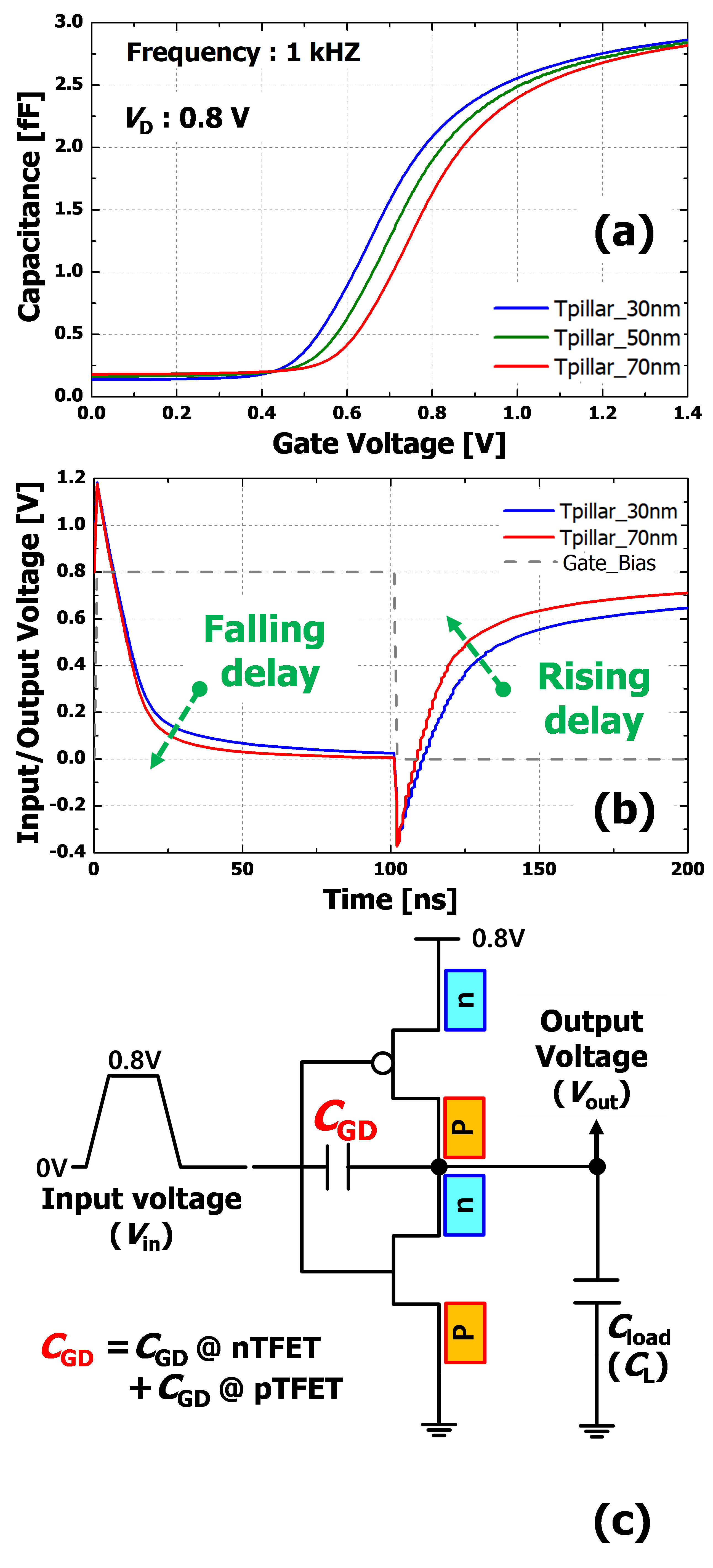## IV. CONCLUSIONS

Mixed-mode device and circuit TCAD simulations are performed to investigate the effects of various pillar conditions on DC characteristics of TFET$_{\mathrm{VS}}$ devices and AC switching characteristics of TFET$_{\mathrm{VS}}$ inverters. As 1) the T$_{\mathrm{pillar}}$ is thicker, 2) the T$_{\mathrm{Si}}$ is increased, 3) the L$_{\mathrm{gate}}$ is longer, and 4) the doping concentration of the pillar is reduced, the I$_{\mathrm{D}}$ is increased in both the transfer and output characteristics due to the stronger influence of V$_{\mathrm{D}}$ on the tunneling barrier between source and channel. Moreover, the V$_{\mathrm{G}}$ controllability on the drain-side channel is verified by C$_{\mathrm{GD}}$-V$_{\mathrm{G}}$ characteristics of TFET$_{\mathrm{VS}}$. As a result, it is found that the weaker V$_{\mathrm{G}}$ controllability on the drain-side channel causes the positive-shifted C$_{\mathrm{GD}}$-V$_{\mathrm{G}}$ curve. Through the transient responses of TFET$_{\mathrm{VS}}$ inverters with various pillar conditions, the effects of the increased I$_{\mathrm{D}}$ and the positive-shifted C$_{\mathrm{GD}}$-V$_{G}$ curve caused by the weaker V$_{\mathrm{G }}$controllability on AC switching performances are investigated thoroughly. Expectedly, it is confirmed that the V$_{\mathrm{out}}$ pre-shoot is reduced and the falling/rising delay are improved.

### ACKNOWLEDGMENTS

This work was supported by INHA UNIVERSITY Research Grant and the BK21 FOUR program of the Education and Research Program for Future ICT Pioneers, Seoul National University in 2021.

### REFERENCES

1
Sze S. M., 1969, Physics of Semiconductor Devices, 1st edn, John Wiley2
Reddick W. M., Amaratunga G. A. J., Jul 1995, Silicon surface tunnel transistor, Appl. Phys. Lett., Vol. 67, No. 4, pp. 494-4963
Bhuwalka K. K., Sedlmaier S., Ludsteck A. K., Toksdorf C., Schulz J., I Eisele , Feb 2004, Vertical tunnel field effect transistor, IEEE Trans. Electron Devices, Vol. 51, No. 2, pp. 279-2824
Choi W. Y., Park B.-G., Lee J. D., King Liu T.-J., Aug 2007, Tunneling field effect transistors (TFETs) with subthreshold swing (SS) less than 60 mv/dec, IEEE Electron Device Lett., Vol. 28, No. 8, pp. 743-7455
Kim H. W., Kim J. H., Kim S. W., Sun M. C., Park E. H., Park B.-G., may 2014, Tunneling field-effect transistor with Si/SiGe material for high current drivability, Jpn. J. Appl. Phys., Vol. 53, No. 6s, pp. 06je12-1-06je12-46
Bjork M. T., Knoch J., Schmid H., Riel H., Riess W., May 2008, Silicon nanowire tunneling field-effect transistor, Appl. Phys. Lett., Vol. 92, No. 19, pp. 193 5047
Fulde M., Heigl A., Weis M., Wimshofer M., Arnim K. V., Nirschl Th., Sterkel M., Knoblinger G., Hansch W., Wachutka G., D , Schmitt-Landsiedel , pp 579–584, Fabrication, optimization and application of complementary multiple-gate tunneling FETs, in Proc. 2nd IEEE Int Nanoelectron. Conf., pp. 579-5848
Gandhi R., Chen Z. X., Singh N., Banerjee K., Lee S. J., Apr 2011, Vertical Sinanowire n-type tunneling FETs with low subthreshold swing (≤ 50 mV/ decade) at room temperature, IEEE Electron Device Lett., Vol. 32, No. 4, pp. 437-4399
Kane E. O., 1961, Theory of tunneling, J. Appl. Phys., Vol. 32, No. 1, pp. 83-9110
Koswatta S. O., Lundstrom M. S., Nikonov D. E., Mar 2009, Performance comparison between p-i-n tunneling transistors and conventional MOSFETs, IEEE Trans. Electron Devices, Vol. 56, No. 3, pp. 456-46511
Mookerjea S., Krishnan R., Datta S., Narayan V., Oct 2009, On enhanced Miller capacitance in inter-band tunnel transistors, IEEE Electron Device Lett., Vol. 30, No. 10, pp. 1102-110412
Mookerjea S., Krishnan R., Datta S., Narayanan V., Sep 2009, Effective capacitance and drive current for Tunnel FET (TFET) CV/I estimation, IEEE Trans. Electron Devices, Vol. 56, No. 9, pp. 2092-2098## Author

Junsu Yu received the B.S. degree in Electrical and Computer Engineering from Seoul National University (SNU) in 2019.

He is currently working toward the Ph.D. degree in Electrical and Computer Engineering from SNU, Seoul, Korea.

His recent interests include tunnel FET, synaptic devices, flash memory, ferroelectric devices.

Daewoong Kwon received Ph.D. degrees in Electrical Engineering at Seoul National University (SNU), Seoul, Korea, in 2017.

He was a senior engineer at Samsung Electro-ics from 2005 to 2014.

He was with University of California at Berkeley, USA, in 2017, as a Post-Doctoral Fellow and with Intel, CA, USA, in 2019.

He is currently an Assistant Professor with the Department of Electric Engineering, Inha University, Incheon, Korea.

Byung-Gook Park received the B.S. and M.S. degrees in electronic engineering from Seoul National University (SNU), Seoul, Korea, in 1982 and 1984, respectively, and the Ph.D. degree in electrical engieering from Stanford University, Stanford, CA, USA, in 1990.

In 1994, he joined the School of Electrical Engineering, SNU, as an Assistant Professor, where he is currently a Professor.